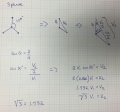# derivation square root of 3 for for delta and wye transformers

#### EL7819

Joined Apr 15, 2011
20
Hello,

I hope I have this in the right forum. I was wondering how mathematicians and or engineers derived the square root of 3 as a constant for delta and wye balanced 3 phase transformers mostly? If not an answer articles to read is fine, anything to point me in the right direction.
My guess would be that there are three
branches and it has something to do with the pythagoreom thereom. I am not sure? If my spelling and or grammer is in some way wrong, I apologize for my insolence.
Thank you, any help is greatly appreciated.

#### steveb

Joined Jul 3, 2008
2,436
My guess would be that there are three
branches and it has something to do with the pythagoreom thereom.
You are close. It is a generalization of that theorem called the cosine law. The three branches imply that the angles between the vectors is 120 degrees, and the cosine law allows the vector length to be calculated for angles other than 90 degrees (which Pythagorus' theorem is limited to).

http://mathworld.wolfram.com/LawofCosines.html

so $$c^2=a^2+b^2-2ab \cos(\theta)$$

becomes

$$c^2=V^2+V^2-2V^2 \cos(120)$$

or

$$c^2=2V^2(1-\cos(120))=3V^2$$

then take the square root of each side to get the following.

$$c=\sqrt{3}V$$

So c represents the line to line voltage Vab or Vbc or Vca, and V represents the phase voltage Va, or Vb or Vc, and the calculation is basically a vector addition (or more accurately vector subtraction) problem.

Last edited:
•#### MrChips

Joined Oct 2, 2009
24,651
$$c=\sqrt{3}V^2$$
Just a minor typo. I know you meant

$$c=\sqrt{3}V$$

#### steveb

Joined Jul 3, 2008
2,436
Just a minor typo. I know you meant

$$c=\sqrt{3}V$$

Thank you!I corrected it. That damn cut and paste operation gets me every time.#### EL7819

Joined Apr 15, 2011
20

#### Georacer

Joined Nov 25, 2009
5,182
@EL7819

You can use the "Thanks" button to show your gratitude towards another member. This way the forum can stay clutter-free.

#### Denny Singleton

Joined May 11, 2016
1
Hello,

I hope I have this in the right forum. I was wondering how mathematicians and or engineers derived the square root of 3 as a constant for delta and wye balanced 3 phase transformers mostly? If not an answer articles to read is fine, anything to point me in the right direction.
My guess would be that there are three
branches and it has something to do with the pythagoreom thereom. I am not sure? If my spelling and or grammer is in some way wrong, I apologize for my insolence.
Thank you, any help is greatly appreciated.@EL7819

You can use the "Thanks" button to show your gratitude towards another member. This way the forum can stay clutter-free.

•GopherT and BR-549